Research papers and code for "Himanshu Asnani":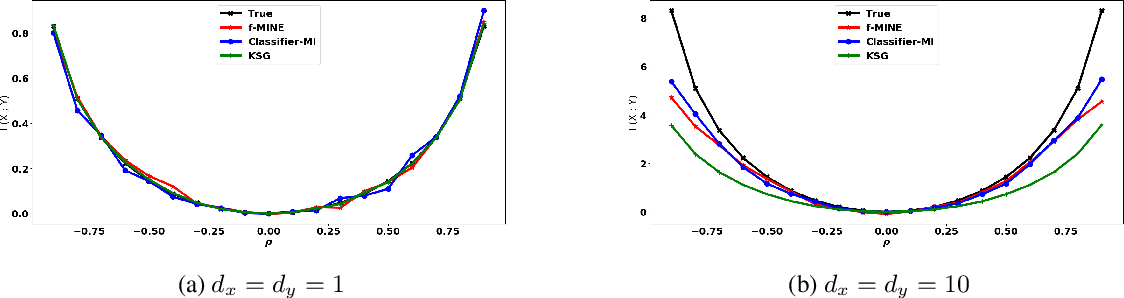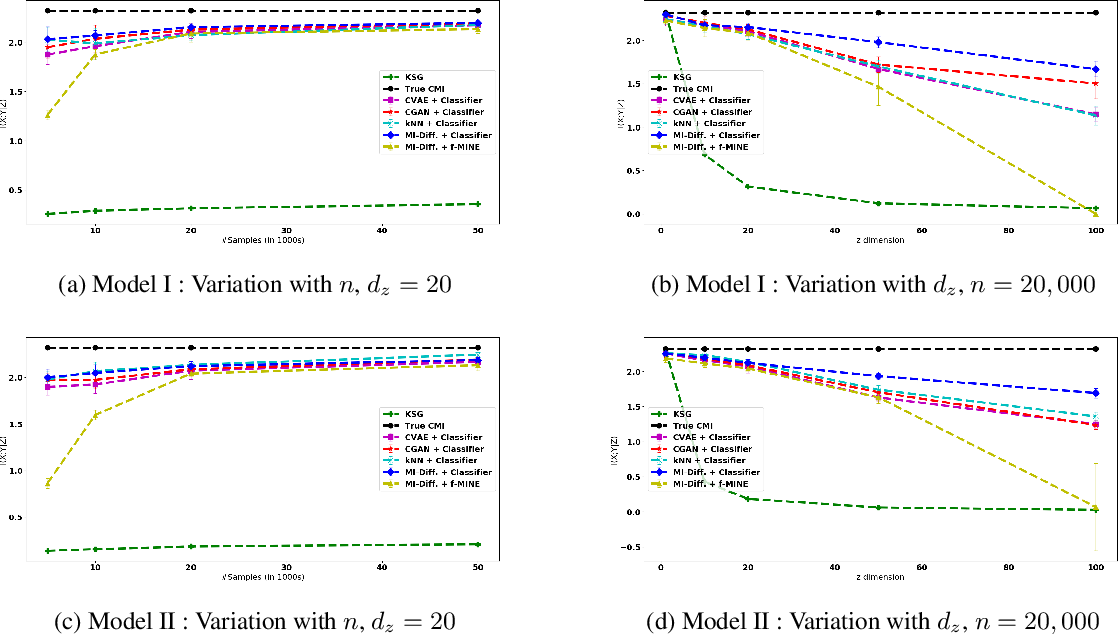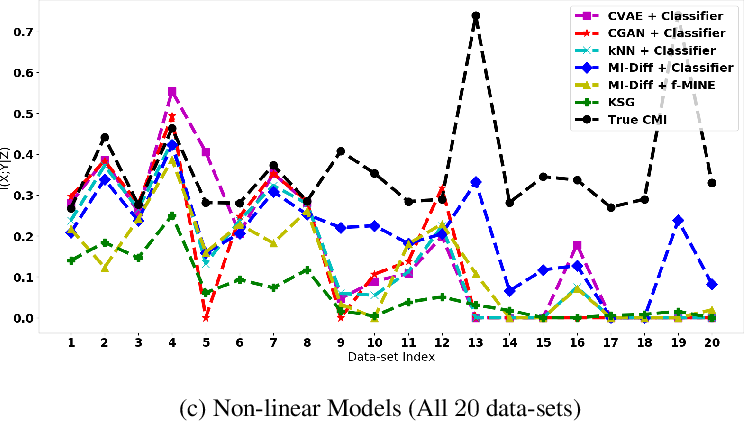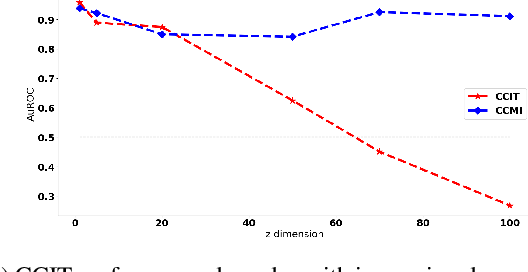Conditional Mutual Information (CMI) is a measure of conditional dependence between random variables X and Y, given another random variable Z. It can be used to quantify conditional dependence among variables in many data-driven inference problems such as graphical models, causal learning, feature selection and time-series analysis. While k-nearest neighbor (kNN) based estimators as well as kernel-based methods have been widely used for CMI estimation, they suffer severely from the curse of dimensionality. In this paper, we leverage advances in classifiers and generative models to design methods for CMI estimation. Specifically, we introduce an estimator for KL-Divergence based on the likelihood ratio by training a classifier to distinguish the observed joint distribution from the product distribution. We then show how to construct several CMI estimators using this basic divergence estimator by drawing ideas from conditional generative models. We demonstrate that the estimates from our proposed approaches do not degrade in performance with increasing dimension and obtain significant improvement over the widely used KSG estimator. Finally, as an application of accurate CMI estimation, we use our best estimator for conditional independence testing and achieve superior performance than the state-of-the-art tester on both simulated and real data-sets.

* Mutual Information and Conditional Mutual Information estimation; Conditional Independence Testing; Classifier two-sample likelihood ratio
Click to Read Paper and Get Code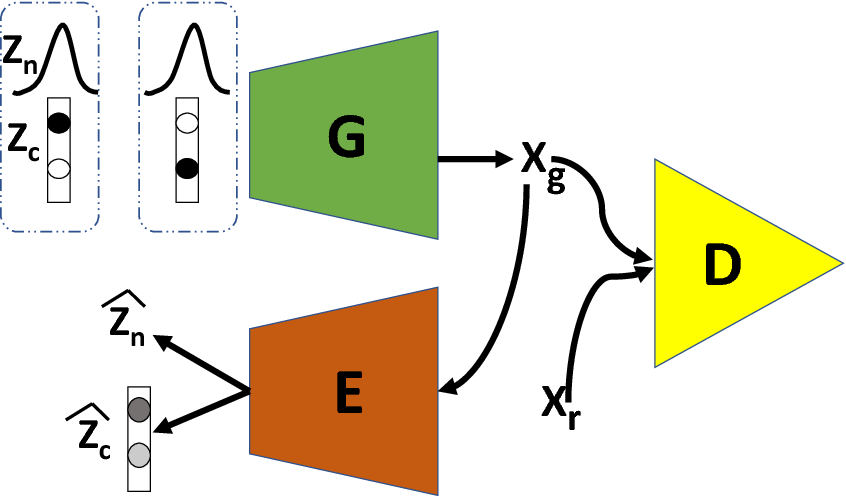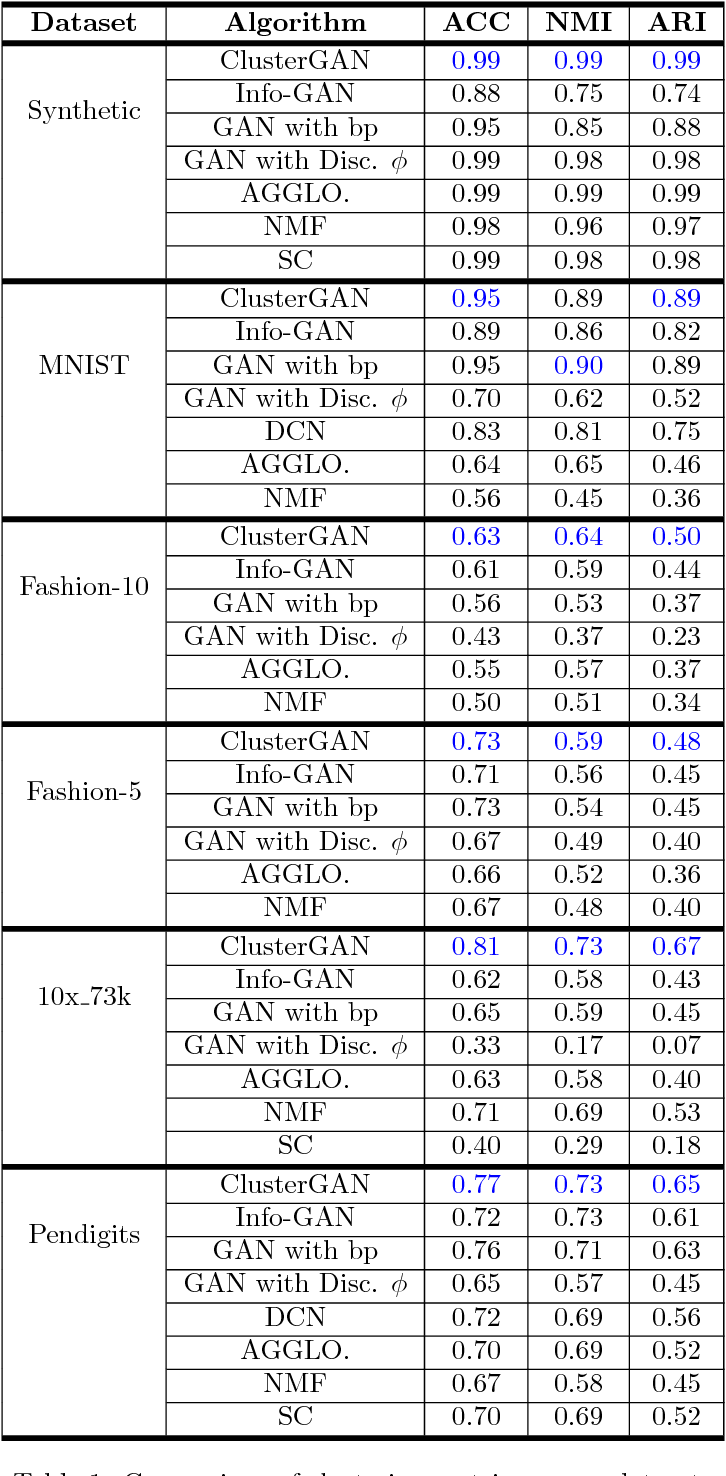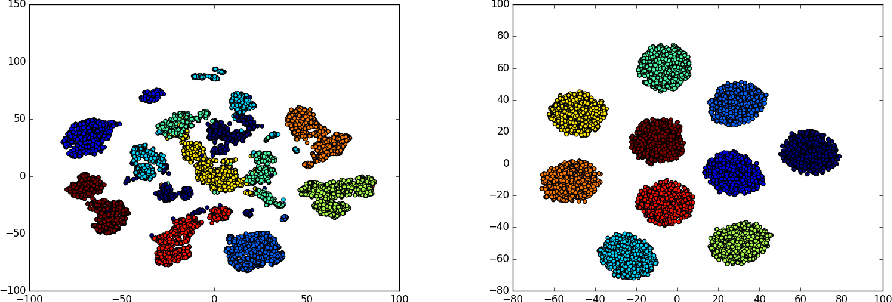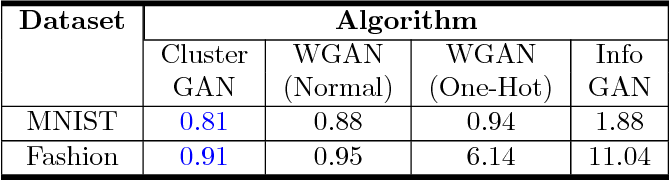Generative Adversarial networks (GANs) have obtained remarkable success in many unsupervised learning tasks and unarguably, clustering is an important unsupervised learning problem. While one can potentially exploit the latent-space back-projection in GANs to cluster, we demonstrate that the cluster structure is not retained in the GAN latent space. In this paper, we propose ClusterGAN as a new mechanism for clustering using GANs. By sampling latent variables from a mixture of one-hot encoded variables and continuous latent variables, coupled with an inverse network (which projects the data to the latent space) trained jointly with a clustering specific loss, we are able to achieve clustering in the latent space. Our results show a remarkable phenomenon that GANs can preserve latent space interpolation across categories, even though the discriminator is never exposed to such vectors. We compare our results with various clustering baselines and demonstrate superior performance on both synthetic and real datasets.

* GANs, Clustering, Latent Space, Interpolation
Click to Read Paper and Get Code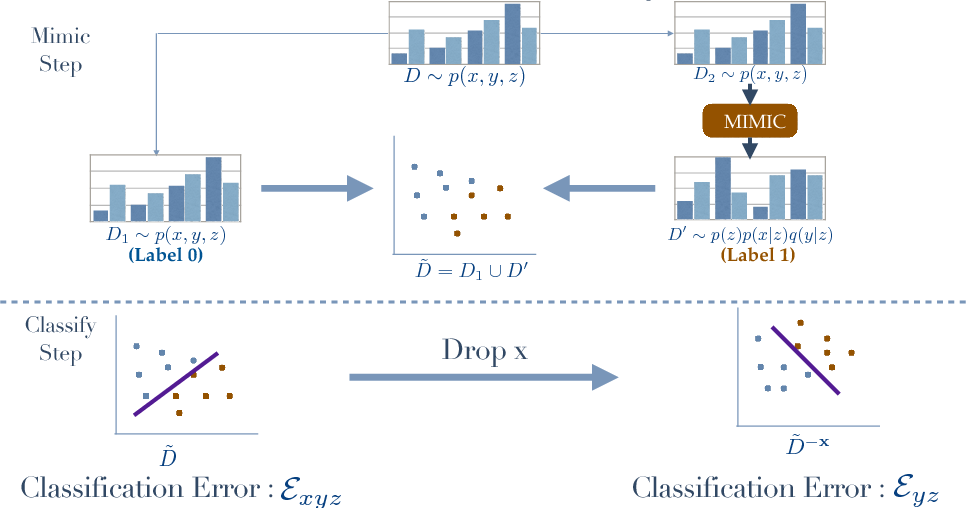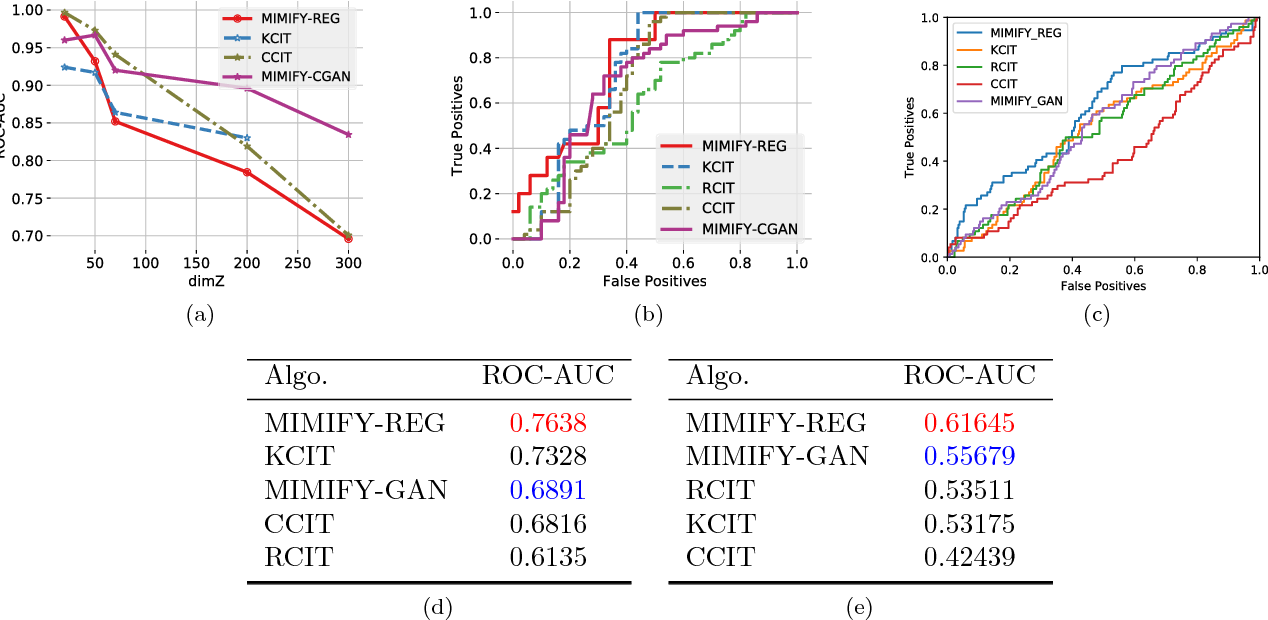Given independent samples generated from the joint distribution $p(\mathbf{x},\mathbf{y},\mathbf{z})$, we study the problem of Conditional Independence (CI-Testing), i.e., whether the joint equals the CI distribution $p^{CI}(\mathbf{x},\mathbf{y},\mathbf{z})= p(\mathbf{z}) p(\mathbf{y}|\mathbf{z})p(\mathbf{x}|\mathbf{z})$ or not. We cast this problem under the purview of the proposed, provable meta-algorithm, "Mimic and Classify", which is realized in two-steps: (a) Mimic the CI distribution close enough to recover the support, and (b) Classify to distinguish the joint and the CI distribution. Thus, as long as we have a good generative model and a good classifier, we potentially have a sound CI Tester. With this modular paradigm, CI Testing becomes amiable to be handled by state-of-the-art, both generative and classification methods from the modern advances in Deep Learning, which in general can handle issues related to curse of dimensionality and operation in small sample regime. We show intensive numerical experiments on synthetic and real datasets where new mimic methods such conditional GANs, Regression with Neural Nets, outperform the current best CI Testing performance in the literature. Our theoretical results provide analysis on the estimation of null distribution as well as allow for general measures, i.e., when either some of the random variables are discrete and some are continuous or when one or more of them are discrete-continuous mixtures.

* 16 pages, 2 figures
Click to Read Paper and Get Code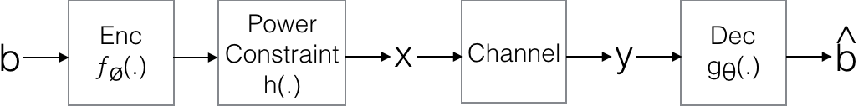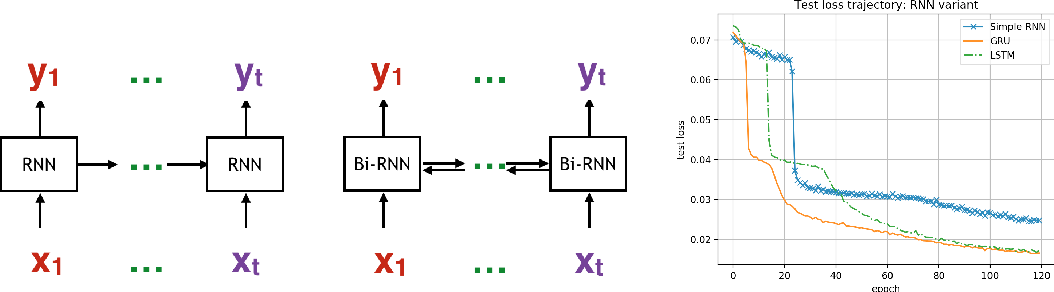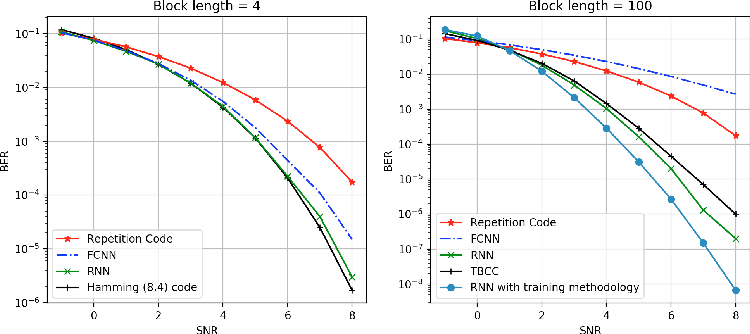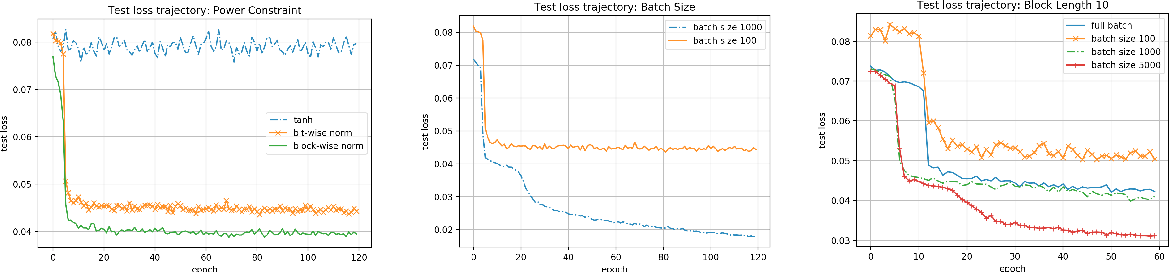Designing channel codes under low latency constraints is one of the most demanding requirements in 5G standards. However, sharp characterizations of the performances of traditional codes are only available in the large block-length limit. Code designs are guided by those asymptotic analyses and require large block lengths and long latency to achieve the desired error rate. Furthermore, when the codes designed for one channel (e.g. Additive White Gaussian Noise (AWGN) channel) are used for another (e.g. non-AWGN channels), heuristics are necessary to achieve any nontrivial performance -thereby severely lacking in robustness as well as adaptivity. Obtained by jointly designing Recurrent Neural Network (RNN) based encoder and decoder, we propose an end-to-end learned neural code which outperforms canonical convolutional code under block settings. With this gained experience of designing a novel neural block code, we propose a new class of codes under low latency constraint - Low-latency Efficient Adaptive Robust Neural (LEARN) codes, which outperforms the state-of-the-art low latency codes as well as exhibits robustness and adaptivity properties. LEARN codes show the potential of designing new versatile and universal codes for future communications via tools of modern deep learning coupled with communication engineering insights.

Click to Read Paper and Get Code